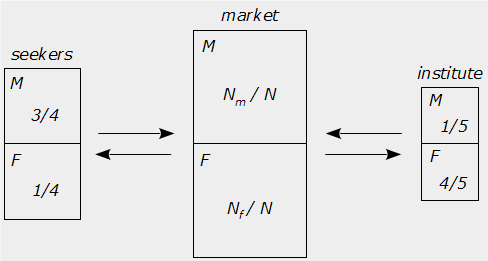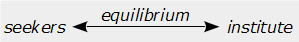## Sunday, 20 April 2008

### Measuring Gender Bias and Prejudice in U.K. Employment

James McComb

Updated March 2013.

If men and women were functionally equal, and there were no gender bias, then the relative proportion of men to women, in a 'gender neutral' workplace, should be similar; or at least reflect the numbers within the pool of available workers. Since bias effects these proportions, then, by induction, a simple measure of the ratio of men to women in the job market, compared to that of a particular employer, should reveal any bias inherent in the selection processes made by that employer or institute.

Poker Die

A perfect none loaded poker die will have, by symmetry, equal probability of landing on any of the six faces; this can then be considered an ideal probability based on logic (deduction). An imperfect or loaded die might, for example, score an Ace more often than any of the other faces; then we would measure the probability by repeated throws of this die until the real probabilities for each face are known, based on empirical measurement (induction), thus:

Ideal probability calculated by symmetry:        $$P_{i}=\dfrac{1}{n}$$

Real probability measured experimentally:        $$P_{r}=\dfrac{score}{throws}$$

The difference between the measured real results and the logical ideal results is the bias; in this case the physical imperfection or loading of the die. Hence:

$$BP_{i}=P_{r}-P_{i}$$

Therefore:

\begin{equation}(B+1)P_{i}=P_{r}\tag{eq.1}\end{equation}

Bias, it should be noted, can be positive or negative with respect to the real result of a particular outcome, and varies between the maximum limits of ±100%; for the example above we would conclude that bias is positive for the Ace, and by complement, negative for the other faces of the loaded die; that is:

\begin{equation}P_{A}+P_{K}+P_{Q}+P_{J}+P_{Ten}+P_{Nine}=1\tag{eq.2}\end{equation}

Note that $$\text{(eq.2)}$$ is true for all cases, both real and ideal. Substituting the real probability from $$\text{(eq.1)}$$ into $$\text{(eq.2)}$$ we get:

\begin{multline}P_{i}(B_{A}+1)+P_{i}(B_{K}+1)+P_{i}(B_{Q}+1)+\cdots\\\\\cdots +P_{i}(B_{J}+1)+P_{i}(B_{Ten}+1)+P_{i}(B_{Nine}+1)=1\end{multline}

Since,$$P_{i}=\frac{1}{6}$$ then:

\begin{equation}B_{A}+B_{K}+B_{Q}+B_{J}+B_{Ten}+B_{Nine}=0\tag{eq.3}\end{equation}

$$\text{(Eq.3)}$$ can be regarded as ‘taking from Peter to give to Pauline’, and reflects the conservative nature of the complementary rule.

Equilibrium Bias

Now let us consider the job market and any bias that may occur according to gender; here the deduction or induction of probabilities, ideal or real, will be represented by the ratios of the proportions by gender in each vocation. The job market for the Isle of Wight is in the order of tens of thousands, the jobseekers divided by gender are approximately 3000 male verses 1000 female, and let us compare an institute of about 300 workers with a ratio of 80% female to 20% male. These are sufficiently large numbers to avoid error by ‘small number’ fluctuation.

If these proportions do not change over time, for example some years, then the system can be regarded as being in equilibrium. That is to say that the rate of male to female employment must equal the rate of male to female unemployment between jobseekers and the job market; otherwise if one rate were higher or lower than the other, then the numbers and proportions would change correspondingly until a new equilibrium position is established.By analogy with the postulate of the ‘zeroth law of thermodynamics’: Two systems in thermal equilibrium with a third are in thermal equilibrium with each other; and relating the thermal bias of temperature with the gender bias of employment, such that:

Implies that:The rate of male to female employment into the institute is presumed to be proportional to the product of the real jobseekers gender ratio and that of the institutes. But since gender bias is suspected of the institute, we replace the institutes exhibited proportions with the ideal proportions claimed by that institute, modified by the gender bias from $$\text{(eq.1)}$$:

$$\left[\dfrac{M}{F}\right]_{seekers}\times\left[\dfrac{(B_m+1)M_i}{(B_f+1)F_i}\right]_{institute}\propto \text{Rate of employment, male per female.}$$

Whereas the rate of unemployment from the institute will simply be a function of the real ratio of those already employed, since the jobseekers do not choose who is removed:

$$\left[\dfrac{M}{F}\right]_{institute}\propto \text{Rate of unemployment, male per female.}$$

Where:

\begin{array}{}M&=&\text{number of males}\\F&=&\text{number of females}\\M_i&=&\text{ideal proportion of males}\\F_i&=&\text{ideal proportion of females}\\B_m&=&\text{bias towards or against males}\\B_f&=&\text{bias towards or against females}\end{array}

At equilibrium, rates are equal, and assuming any constants of proportionality resolve to unity for dimensionless ratios, then:

$$\left[\dfrac{M}{F}\right]_{seekers}\times\left[\dfrac{(B_m+1)M_i}{(B_f+1)F_i}\right]_{institute}= \left[\dfrac{M}{F}\right]_{institute}$$

Rearranging:

\begin{equation}\left[\dfrac{(B_m+1)M_i}{(B_f+1)F_i}\right]_{institute}=\dfrac {\left[M/F\right]_{institute}}{\left[M/F\right]_{seekers}}\tag{eq.4}\end{equation}

If the institute claimed zero gender bias, then $$B_m=B_f=0$$, and substituting values from the job market into $$\text{(eq.4)}$$, would yield:

$$\left[\dfrac{M_i}{F_i}\right]_{institute}=\dfrac {\left[M/F\right]_{institute}}{\left[M/F\right]_{seekers}}=\dfrac {\left[1/4\right]_{institute}}{\left[3/1\right]_{seekers}}=\dfrac{1}{12}$$

That is to say, that if the institute claimed zero gender bias, then they would have to show that the female application rate $$A_{(m/f)}$$, was 12 times more than that of males; alternatively they would have to show that women were more competent at the job, and having 12 times more skill $$S_{(m/f)}$$, or some combination thereof.

On the other hand, if the institute insists that there is equal gender skill, and application rates, then $$M_i=F_i$$, and $$\text{(eq.4)}$$ would simplify to:

$$\left[\dfrac{(B_m+1)}{(B_f+1)}\right]_{institute}=\dfrac {\left[M/F\right]_{institute}}{\left[M/F\right]_{seekers}}=\dfrac {\left[1/4\right]_{institute}}{\left[3/1\right]_{seekers}}=\dfrac{1}{12}$$

Solving for bias, and using the relationship $$B_m+B_f=0$$, from $$\text{(eq.3)}$$, the complementary principle, gives the results for the individual gender bias inherent in the institute:

$$B_m=\dfrac{-11}{13}$$

$$B_f=\dfrac{+11}{13}$$

If all else were equal, then this institute would be branded biased against males, and for females. They would need to explain the blatant contradiction within their policies of 'equal opportunity', to account for $$M_i\neq F_i$$, The claim that the institute practices 'gender equality', is therefore bogus. Those responsible have transgressed the 'equal opportunities' law; unless this law is itself bogus, and blatantly biased against Wightmen!

Snow White and the Seven Dwarves

In the politically synthetic world of presumed equality, and suspected bias, it is possible to derive equations of probability for an individual male or female in situations where there are admixtures of the number of males $$M$$ and females $$F$$, applying for the same job.

Firstly, introducing and defining $$J_{(m/f)}$$: as the prejudice coefficient, by consolidating the individual gender bias terms of $$\text{(eq.4)}$$, into a single term for both genders:

\begin{equation}J_{(m/f)}=\dfrac{B_m+1}{B_f+1}\tag{eq.5}\end{equation}

The prejudice coefficient $$J_{(m/f)}$$, varies between 0 and , with 1 representing zero bias. Values less than 1 indicate prejudice against males, and values larger than 1, imply prejudice against females. This hyperbolic coefficient is reciprocal to $$J_{(f/m)}$$.

Secondly, solving for bias in $$\text{(eq.1)}$$, and substituting proportions for probabilities, we induce:

$$J_{(m/f)}=\dfrac{B_m+1}{B_f+1}=\dfrac{M/M_i}{F/F_i}$$

For pure bias we assume $$M_i=F_i$$, therefore the prejudice coefficient becomes the ratio of gender probabilities, derived by induction from the institute and seeker proportions respectively:

\begin{equation}J_{(m/f)}=\dfrac{[M]_{institute}/[M]_{seekers}}{[F]_{institute}/[F]_{seekers}}=\dfrac{P_m}{P_f}\tag{eq.6}\end{equation}

Note that the middle expression of $$\text{(eq.6)}$$ is the same as the right hand side of $$\text{(eq.4)}$$, except for the rearrangement of numerator and denominator in regard to gender rather than vocation.

The equation of gender probabilities for $$M$$ males and $$F$$ females, is derived from $$\text{(eq.2)}$$:

$$M.P_m+F.P_f=1$$

Substituting $$P_f$$ from $$\text{(eq.6)}$$:

$$M.P_m+F.\left[\dfrac{P_m}{J_{(m/f)}}\right]=1$$

Solving for the male probability yields:

\begin{equation}P_{m}=\dfrac{1}{M+F.(J_{m/f})^{-1}}\tag{eq.7a}\end{equation}

And by symmetry, the female probability:

\begin{equation}P_{f}=\dfrac{1}{F+M.(J_{m/f})}\tag{eq.7b}\end{equation}

Example 1:

When applying to the prejudiced institute in the section above, with $$F=1$$ Snow White, verses $$M=7$$ Dwarves, there would be the following probabilities of getting the job:

Snow White's probability $$\text{(eq.7b)}$$:                    $$P_{f}=\dfrac{12}{19}\approx \dfrac{2}{3}$$

Probability for one of the Dwarves $$\text{(eq.7a)}$$:        $$P_{m}=\dfrac{1}{19}$$

Example 2:

For the situation of $$F=7$$ Snow Whites, verses $$M=1$$ Dwarf, at the interview:

One of the Snow Whites $$\text{(eq.7b)}$$:                     $$P_{f}=\dfrac{12}{85}\approx \dfrac{1}{7}$$

The Dwarf's probability $$\text{(eq.7a)}$$:                        $$P_{m}=\dfrac{1}{85}$$

Value, Prejudice, Application Rate, and Skill

In the real and natural world $$M_i\neq F_i$$, since men and women have evolved to be functionally different, therefore an institute may judge the value of its employees based on a combination of both gender skill and bias. The gender ratio of employees reflects on this 'judged' value held by the institute, via the relationship of gender proportions of job seekers:

$$\left[\dfrac{M}{F}\right]_{seekers}\propto\left[\dfrac{M}{F}\right]_{institute}$$

The constant of proportionality is the value given to males per female $$V_{(m/f)}$$, imposed by the institute when selecting employees:

\begin{equation}V_{(m/f)}.\left[\dfrac{M}{F}\right]_{seekers}=\left[\dfrac{M}{F}\right]_{institute}\tag{eq.8}\end{equation}

Solving for $$V_{(m/f)}$$, we get the ratio of ratios, [c.f. $$\text{(eq.4)}$$]:

\begin{equation}V_{(m/f)}=\dfrac{[M/F]_{institute}}{[M/F]_{seekers}}\tag{eq.9}\end{equation}

The value is measurable by the ratios of the job market; by intuition, it is composed of at least three elements: prejudice $$J_{(m/f)}$$, application rate $$A_{(m/f)}$$, and skill $$S_{(m/f)}$$; it is therefore proposed that:

\begin{equation}V_{(m/f)}=J_{(m/f)}.A_{(m/f)}.S_{(m/f)}\tag{eq.10}\end{equation}

Application rate $$A_{(m/f)}$$, is simply the ratio of numbers of males per female that have applied for a particular position:

\begin{equation}A_{(m/f)}=\left[\dfrac{M}{F}\right]_{\text{number of applications}}\tag{eq.11}\end{equation}

Naturally, we should recognise variation in gender competence for specific types of job, as possibly measured by test scores in entrance exams, or past proven experience, such as dwarves make better diamond miners, whilst Snow White may make a better gold digger, and replace the ideal proportions with those based on measured merit, or skill for that job:

\begin{equation}S_{(m/f)}=\left[\dfrac{M}{F}\right]_{\text{competence scores}}\tag{eq.12}\end{equation}

Prejudice $$J_{(m/f)}$$, has already been introduced in $$\text{(eq.5)}$$ above, and now we can include the rearranged solution of $$\text{(eq.10)}$$, thus:

\begin{equation}J_{(m/f)}=\left[\dfrac{B_m+1}{B_f+1}\right]_{institute}=\dfrac{V_{(m/f)}}{A_{(m/f)}.S_{(m/f)}}\tag{eq.13}\end{equation}

Hence, with $$\text{(eq.9)}$$ and $$\text{(eq.13)}$$, for any institute, given the gender ratios of the job market, application rates, and any competency test scores measuring skill, we can determine the value, bias, and prejudice, held by that institute, regarding the selection of males and females for employment.

This shows that any effort made to address perceived prejudice in the work place, by quota filling towards desired gender ratios, thus artificially adjusting $$V_{(m/f)}$$, must lead to real prejudice, and ultimately the corruption of value and skill in the workplace. Indeed, the feminist agenda encourages this cultural conceit, similar to a social variation of the 'Dunning-Kruger Effect'.

Example 3:

Now if skill and application rates were equal for men and women, then according to $$\text{(eq.13)}$$, the prejudice of the institute would simply equal the value imposed by the institute, as in the previous section. So let us suppose that women were somewhat more virtuous than men for the sake of this example, and say that women's application rates were 3 times more than men's; and that their skill scores in pertinent tests, gave 2 times as many women passes than the men achieved. Using the market numbers from the previous section, and applying our fantasy values of women's virtue, we still get a measure of prejudice against men of 2 to 1:

$$J_{(m/f)}=\dfrac{[1/12]_{(m/f)}}{[1/3]_{(m/f)}.[1/2]_{(m/f)}}=\left[\dfrac{1}{2}\right]_{(m/f)}$$

Example 4:

And of course, if men were the virtuous ones, which applied 3 times more often, and passed 2 times more often in pertinent tests, then the prejudice against men would be 72 times that of women, in order to achieve the same job market figures!:

$$J_{(m/f)}=\dfrac{[1/12]_{(m/f)}}{[3/1]_{(m/f)}.[2/1]_{(m/f)}}=\left[\dfrac{1}{72}\right]_{(m/f)}$$

If this analysis is true, and the typical proportions exhibited by the job market are as estimated, then justice must be done to right this great wrong against Wightmen. The State must acknowledge the Marxist-Feminist dominated public sector, which is responsible for this blatant embezzlement of gainful employment from Wightmen.

Further, any demeaning exercises to 'improve the employability' of Wightmen, such as the mandatory 'Employment Training Programme', will not solve the inherent problem of misandric prejudice; since any gains of virtue by an unemployed Wightman can, and probably will, be countered by a proportional increase of the bias and prejudice lined against employing him.

Here is an MP3 recording of the last 11 minutes of a job interview at the DWP